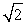# [Solved] If the Absolute Temperature of a Gas Is Doubled,what Is

Question 55
Multiple Choice

## If the absolute temperature of a gas is doubled,what is the change in the average kinetic energy of its molecules?

A)no change
B)increases by a factor of 2
C)decreases by a factor of 2
D)increases by a factor ofE)decreases by a factor of10+ million students use Quizplus to study and prepare for their homework, quizzes and exams through 20m+ questions in 300k quizzes.

### Physics & Astronomy

Explore our library and get Introduction to Physical Science Homework Help with various study sets and a huge amount of quizzes and questions

120

Study sets

3.1K

Quizzes

233.2K

Questions

Upload material to get free accessInvite a friend and get free accessSubscribe and get an instant access